Subsections

# Flory Theory

## Approximation

Lattice gas analogy--we have N cells, Np particles A and N-Np particles B. We will use canonical ensemble (Ising model for magnetics corresponds to grand canonical ensemble!). Partition function:(1)
Flory idea: substitute (1) by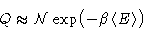## Free Energy

The number of terms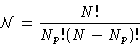Average energy: we have Np As. Each A has z neighbors. About Npz/N of them are A, (N-Np)z/N are B. We have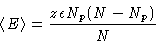Free energy: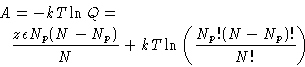(2)

Stirling formula: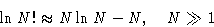New variables: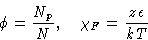Free energy: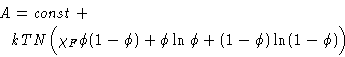(3)

## Critical Point

System is symmetric--critical point is at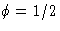. We obtain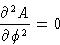or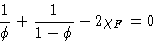which gives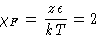The system is mixed above critical point and demixed below it

## Binodal & Spinodal

Free energy of Flory system below critical point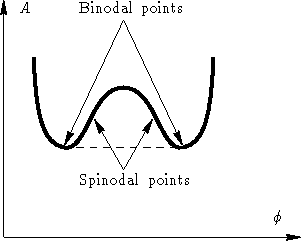On spinodal the second derivative is zero:On binodal there is local minimum--first derivative is zero: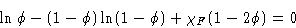In critical point binodal & spinodal coincide: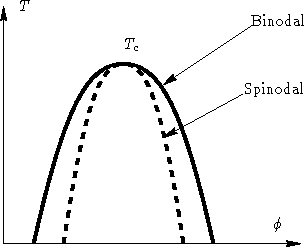## Problems of Flory Theory

1.
It is not clear how to calculate next approximation(s)
2.
Works because of compensation of errors:
• We overestimate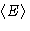: correlations decrease average energy
• We overestimate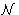: most of states have too small contribution to Q
Flory theory overestimates both energy and entropy--the resulting expression is more or less'' right.Next: Self-Consistent Field Theory Up: Mean Field Approach Previous: General Idea

© 1997 Boris Veytsman and Michael Kotelyanskii
Tue Oct 14 22:58:59 EDT 1997